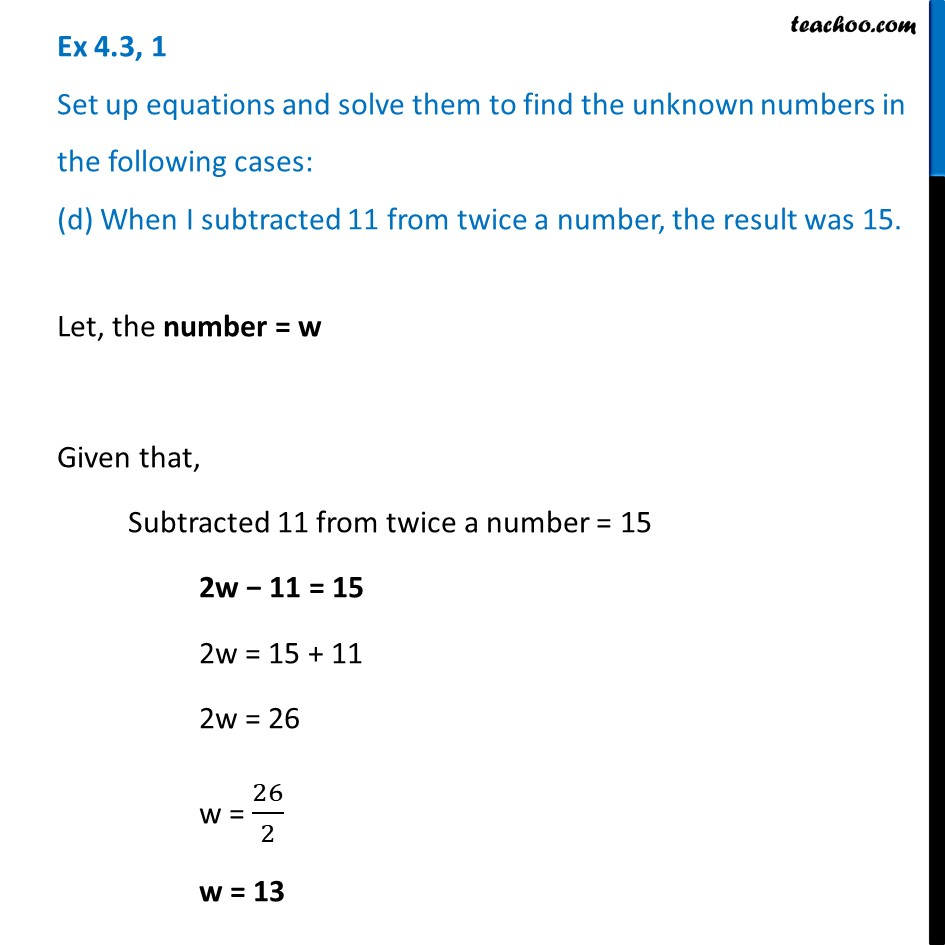Ex 4.3

Chapter 4 Class 7 Simple Equations
Serial order wiseLearn in your speed, with individual attention - Teachoo Maths 1-on-1 Class

### Transcript

Ex 4.3, 1 Set up equations and solve them to find the unknown numbers in the following cases: (d) When I subtracted 11 from twice a number, the result was 15.Let, the number = w Given that, Subtracted 11 from twice a number = 15 2w − 11 = 15 2w = 15 + 11 2w = 26 w = 26/2 w = 13